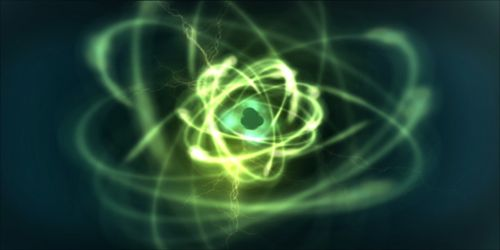# Atomic Energy

Atomic Energy:

In 1905 famous scientist Albert Einstein showed that matter and energy were actually identical. Matter can be transformed into energy. If a substance of mass m is completely transformed into energy, the amount of energy obtained is, E = mc2, here ‘c’ is the speed of light = 3 x 108 ms-1.

This is called the Einstein’s mass-energy equivalent lass.

Suppose the mass of a substance is 1 kg. If this substance can be converted into energy, then the available energy will be, E = 1 x (3 x 108)2 = 9 x 1016 J. Because of this intrinsic proportionality between mass and energy, mass and energy of matter are equivalent and they are two different forms of the same material.

By disintegrating a nucleus of by combining two light nuclei into one enormous energy is available. Atomic energy may include:

• Nuclear binding energy, the energy required to split a nucleus of an atom.
• Nuclear potential energy, the potential energy of the particles inside an atomic nucleus.
• Nuclear reaction, a process in which nuclei or nuclear particles interact, resulting in products different from the initial ones; see also nuclear fission and nuclear fusion.
• Radioactive decay, the set of various processes by which unstable atomic nuclei emit subatomic particles.

The name of this energy is nuclear energy. But apparently, it is called atomic energy. Energy can be produced from an atom by two processes. These are (1) Nuclear fission and (2) Nuclear fusion.